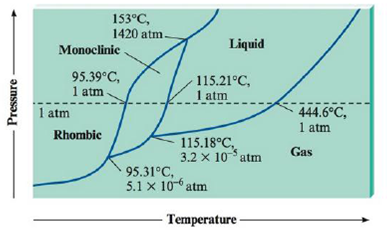Chapter 10, Problem 106E

Chapter
Section
Textbook Problem

Sulfur exhibits two solid phases, rhombic and monoclinic. Use the accompanying phase diagram for sulfur to answer the following questions. (The phase diagram is not to scale.)a. How many triple points are in the phase diagram?b. What phases are in equilibrium at each of the triple points?c. What is the stable phase at 1 atm and 100.°C?d. What are the normal melting point and the normal boiling point of sulfur?e. Which is the densest phase?f. At a pressure of 1.0 × 10-5 atm, can rhombic sulfur sublime?g. What phase changes occur when the pressure on a sample of sulfur at 100.°C is increased from 1.0 × 10−8 atm to 1500 atm?

Interpretation Introduction

Interpretation: The following statements to be explained by using given phase diagram of Sulphur.

Concept Introduction

Phase diagram: Under different temperature and pressure, physical states of the substance that are represented in graph are known as phase diagram. The temperature represents in X-axis and pressure in Y-axis.

Triple point: For given substance, three phases (solid, liquid and gas) are coexisting in thermodynamic equilibrium at particular temperature and pressure.

Critical point: In a phase diagram, a point at which both liquid and gaseous state of the substance having indistinguishable density.

Normal melting point: Normal melting point of the solids defined as the temperature at which solid melts at 1 atmospheric pressure. Normal melting point is differing from melting point in which pressure would be defined.

Normal boiling point: Normal boiling point of the liquid is defined as the temperature at which liquid boils at 1 atmospheric pressure. Normal boiling point is differing from boiling point in which pressure would be defined.

Explanation

Explanation

a)

Record the given data

The given phase diagram for Sulphur was recorded as shown.

Three triple points are present in phase diagram. These are,

1) Rhombic, monoclinic and gas

2) Monoclinic, liquid and gas

3) Monoclinic, liquid and rhombic

b)

In the given phase diagram of Sulphur, in which the triple points at the temperature of 95.31°C are Rhombic, monoclinic and gas. At 115.18°C are monoclinic, liquid and gas and at 153°C are monoclinic, liquid and rhombic.

c)

From phase diagram Monoclinic solid phase is stable point at 100°C and 1atm.

d)

The normal melting point of Sulphur is 115.21°C because which is appears in liquid phase at I atm and normal boiling point of Sulphur is 444.6°C because it is appears in gas phase at 1 atm.

e)

From phase diagram Rhombic is the densest phase, because the monoclinic-rhombic equilibrium line has a positive slope, and the solid-liquid equilibrium lines also has positive slopes.

f)

Rhombic sulphur cannot sublime at pressure of 1.0×105. P = 1.0 × 10-5 atm is at a pressure  between 95

Still sussing out bartleby?

Check out a sample textbook solution.

See a sample solution

The Solution to Your Study Problems

Bartleby provides explanations to thousands of textbook problems written by our experts, many with advanced degrees!

Get Started